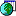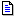﻿ Convertidor de formato IEEE 754
﻿

Disponible la nueva versión "donationware" 7.3 de OrganiZATOR
Descubre un nuevo concepto en el manejo de la información.
La mejor ayuda para sobrevivir en la moderna jungla de datos la tienes aquí.

Curso C++

 [Home]  [Inicio]  [Índice]

#### Convertidor de formato

Nota: la versión que aquí se reproduce, con autorización y por cortesía del Dtr Christopher Vickery, del departamento de Ciencias de la Computación del Queens College de NY , es una traducción al español del original (http://babbage.cs.qc.edu/courses/cs341/IEEE-754.html).

##### §1 Presentación

A continuación se incluye un convertidor automático de formato que permite introducir un número en formato decimal, y obtener el aspecto de su almacenamiento binario (en simple y doble precisión) según el Estándar IEEE 754.  El convertidor proporciona también dos resultados adicionales, son las representaciones hexadecimal (2.2.4b) y decimal de la representación binaria IEEE 754 del número introducido .

Su funcionamiento es muy sencillo: Introducir el número en la casilla correspondiente en formato decimal (puede utilizarse también notación científica). A continuación pulsar el botón correpondiente al tipo de conversión solicitada .

Nota: observe que en campo "significando" incluye la representación de los bits 0-22 o 0-51 (según el caso) y además se ha incluido el bit-j que no forma parte de la representación IEEE 754 (este bit se supone implícito).

 Número a convertir:

Valor decimal del número introducido:

Representación binaria en simple precisión (32 bits):

Tipo:
 Bit 31 Signo (0=+/1=-) Bits 30 - 23 Exponente Valor decimal del exponente y su equivalente - 127 = Bits 22 - 0 Significando Valor decimal equivalente

Representación binaria en doble precisión (64 bits):

Tipo:
 Bit 63 Signo (0=+/1=-) Bits 62 - 52 Exponente Valor decimal y su equivalente - 1023 = Bits 51 - 0 Significando Valor decimal equivalenteInicio.

 Tenga en cuenta que el proceso de conversión a binario para su representación conforme al Estándar IEEE 754, puede implicar un redondeo, por lo que el número obtenido puede no coincidir exactamente con el valor introducido. Por ejemplo, Introduzca el número 7.27E12 (utilizar el punto . para los decimales), y a continuación el botón "Sin redondeo". Observará que la representación binaria en simple precisión equivale a 7.2699997e12, mientras que la de doble precisión si es capaz de representar exactamente el número solicitado (el valor decimal es 7270000000000.0000). En el primer caso, la diferencia es 0.0000003. Si en lugar del botón "Sin redondeo" se solicita la versión redondeada, la representación binaria en doble precisión sigue siendo exacta, mientras que la de precisión simple arroja el valor 7.2700002e12. La diferencia con el valor teórico es ahora 0.0000002 (el redondeo IEEE 754 ha mejorado la precisión del valor almacenado).

 El programa JavaScript es original de Quanfei Wen, un estudiante del Queens College. Posteriormente Kevin J. Brewer añadió diversas modificaciones que mejoraron su conformidad con el Estándar. Los créditos y lista de modificaciones son los siguientes (respetamos el original Inglés):

Modifications by Kevin J. Brewer, 1998/08/20 to 1998/09/21

- Corrected exponential ranges to those specified in IEEE-754.
- Correction of decimal exponent field size.
- Made correction so that decimal significand is displayed even when its value is 0.
- decBinVal array sizes corrected for small values (those near 1.0*2**-126 for 32-bit or 1.0*2**-1022 for 64-bit).
- Corrected ieee32.Convert2Dec() to ieee64.Convert2Dec() in the ieee64 section.
- Added support for zero and denormalized numbers (those less than 1.0*2**-126 for 32-bit or 1.0*2**-1022 for 64-bit).
- Modified significand input field to hold the notationally largest range input value (with the most significant digits, exponent, and signs), -4.94065645841246544E-324.
- Use only 1 set of intBinVal and decBinVal arrays so that 32-bit results will be marked invalid when 64-bit values greater than 3.40282347E38 are entered.
- Joined arrays intBinVal and decBinVal into one array, BinVal, in order to ease the manipulations involved in rounding.
- Added IEEE-754 round-to-nearest value rounding mode.
- Added input echo field which displays how the host machine sees the input value (round-off, number of significant digits, max and min exponentials, etc.).
- For both precisions, added an echo field which displays the input value accurate to the number of bits in that precision's significand.
- Added action so that when an input value overflows a precision, set that precision's outputs to the precision's Infinity values instead of having the bits be replaced by "#"s.
- For both precisions, added a status field indicating from high to low: overflow, normal, denormalized, underflow, and normal for zero.
- Run Convert2Bin() only once per precision (not for each substring).
- Added the ability to round or not to round by providing 2 activation buttons labeled as such.
- Reduced exponent input field to hold up to the notationally largest exponent value (with the most significant digits and sign), -324, unless someone wants to oddly decimalize (add fractional/decimal parts to) the exponent.
- Replaced calls to round() with tests and corrections for rounding up to calls to floor() which always rounds down.

1998/09/28 to 1998/09/30

- Made BinVal external to Convert2Bin (and therefore local to function ieee) as this.BinVal as is required in IEEE-754hex64.html and IEEE-754hex32.html so that Unix 'diff' has some chance at comparing this file with IEEE-754hex64.html or IEEE-754hex32.html .
- General clean-ups.
- Removed the optional "Exponent:" input field due to its problem of causing the entire input to underflow to zero when its value is -324, the problems
encountered when trying to correct this deficiency programatically, and its superfluousness due to the ability of entering scientific notation (4.94065645841246544E-324) into the "Significand:" field alone.
- Renamed the "Significand:" input field to "Decimal Floating-Point:".

1998/10/06 to 1998/10/07

- Added converting all floating-point inputs into canonical form (scientific notation, +x.xxE+xxxx form) with constant exponential width and plus sign usage by adding the Canonical routine, in order to simplify the string number comparisons involved in the 1.7976931348623157E+308 overflow check.
- Found and corrected bug in both binary outputs created by some unknown JavaScript scoping problem for the symbol 'output' by introduction of the Canonical routine (never in a released version).
- Floating-point input is now hand parsed and then its magnitude is compared against +1.7976931348623157E+00308 in the OvfCheck routine. If the magnitude is greater than this value (compared as a string), set all outputs to the appropriate Infinity values. The JavaScript implementation of IEEE-754 64-bit precision clips such values at 1.7976931348623157E+308.
- Greatly improved the efficiency of the Convert2Hex routine.

1998/10/20

- Allow power of 10 indicator in numStrClipOff to be "E" as well as "e" in case not all browsers use "e".
- Reordered 'numerals' in OvfCheck so that their index (value) is the same as that which the numeral represents.

1998/10/23

- With hand parsed floating-point input, compare its magnitude against +2.4703282292062328E-00324 in the UndfCheck routine. If the magnitude is less than this value (compared as a string), set 'this.StatCond' to "underflow". The JavaScript implementation of IEEE-754 64-bit precision underflows such values to zero.
- The above required all settings of 'this.StatCond' to "normal" to have to be removed, "normal" rather than "error" is now the default.
- With hand parsed input, set the output sign bit from its minus sign character before the input is turned into a numeric. This supports input of negative zeros, "-0", and those values which underflow to it.

1998/10/28

- The central testing section of OvfCheck and UndfCheck were joined together as the single routine A_gt_B.
- The translation which moves an input's base 10 exponential (the sign and value to the right of the "E") to its left end as its sign and most significant digits is now performed by the MostSigOrder routine.
- Due to tests now involving negative exponentials in A_gt_B (via UndfCheck), a bias of 50,000 is added to all exponentials, when moved by MostSigOrder, in order to simplify numeric compares performed as iterative comparisons of individual digits in strings.

1998/10/29

- Floating-point input is now hand parsed, manipulated, and placed into the this.BinVal array only once by introduction of the Dec2Bin routine.  As a result, the Convert2Bin routine now used is quite similar to that of IEEE-754hex64.html and IEEE-754hex32.html.

1999/03/04

- Corrected displaying error in numStrClipOff when the number of digits of precision for a particular IEEE-754 format is less than the number of digits returned by the system (before the value is large enough that the system returns it in "E" notation).
Error found by Bill Maxwell (billmax@compuserve.com).

1999/03/05

- The system returns values such as 1.0E21 simply as 1E21. In numStrClipOff, made adjustments to correct the display output when the system returns such values.  Due to the idealized nature of the input to numStrClipOff vs. that of Canonical, many simplifications to the code in numStrClipOff were made.
- Added a Clear button next to the input field which clears the input field and all result fields.

1999/05/17

- Removed <FONT FACE="Arial"> which is not displayed the same by all browser versions.
- Balanced all <FONT> tags with </FONT> tags.
- Removed all value layout comments since that information is much better presented in the IEEE-754references.html file.
- Aligned all "Bit xx" and "Bits xx - xx" headings.
- Replaced all occurrences of the term "unnormalized" with the term "denormalized" preferred by the standard.
- Changed the "Decimal value of the exponent" display from "b + [e] = [f]" to "[f] - b = [e]" where b = 127 or b = 1023
- Headings "Exponent" changed to "Exponent Field" and headings "Decimal value of the exponent" changed to "Decimal value of exponent field and exponent".

1999/05/28

- Rounded the 32-bit decimal significand just like the 32-bit full decimal value.
- Fixed displaying problem in numStrClipOff in which values of 0.000000099... and smaller (in 32-bit) are displayed as 0.0000000 because the values are not small enough for the system to return them in "E" notation and similarly for 0.000000000000000099... and smaller in 64-bit.

 La versión de simple precisión (32-bit) redondeada, utiliza el modo IEEE-754 "round-to-nearest-value".